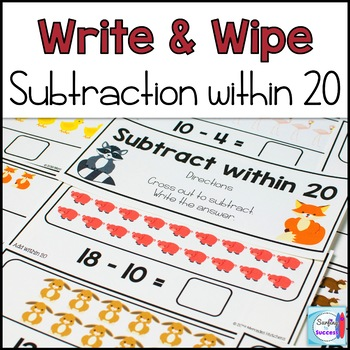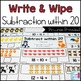# Subtraction Write and Wipe: Subtraction within 20Subject
Resource Type
File Type

PDF

(42 MB|65 pages)
Standards
Also included in:
1. These write and wipe sets make great kindergarten and first grade math centers to help your students become fluent in subtraction. Simply laminate and cut. One set provides pictures and the students cross off to subtract write the difference. The other set provides the equation and students draw t
\$13.00
\$10.40
Save \$2.60
• Product Description
• StandardsNEW
These write and wipe sets make great first grade math centers for subtraction fact fluency. Simply laminate and cut. One set provides pictures and the students cross off to subtract within 20. The other set provides the equation and students draw the pictures and write the difference. The Draw Your Own cards also work great for manipulatives. Students can use manipulatives to represent the problems. Use new manipulatives to keep the center fresh and engaging.

Contains:
• Directions and Tips for Use
• an Animal Themed set
• a 'Draw Your Own' set
Add and subtract within 20, demonstrating fluency for addition and subtraction within 10. Use strategies such as counting on; making ten (e.g., 8 + 6 = 8 + 2 + 4 = 10 + 4 = 14); decomposing a number leading to a ten (e.g., 13 - 4 = 13 - 3 - 1 = 10 - 1 = 9); using the relationship between addition and subtraction (e.g., knowing that 8 + 4 = 12, one knows 12 - 8 = 4); and creating equivalent but easier or known sums (e.g., adding 6 + 7 by creating the known equivalent 6 + 6 + 1 = 12 + 1 = 13).
Fluently add and subtract within 5.
Total Pages
65 pages
N/A
Teaching Duration
1 Year
Report this Resource to TpT
Reported resources will be reviewed by our team. Report this resource to let us know if this resource violates TpT’s content guidelines.